Courses

# Test: Formal Charge & Resonance

## 22 Questions MCQ Test Chemistry for JEE | Test: Formal Charge & Resonance

Description
This mock test of Test: Formal Charge & Resonance for JEE helps you for every JEE entrance exam. This contains 22 Multiple Choice Questions for JEE Test: Formal Charge & Resonance (mcq) to study with solutions a complete question bank. The solved questions answers in this Test: Formal Charge & Resonance quiz give you a good mix of easy questions and tough questions. JEE students definitely take this Test: Formal Charge & Resonance exercise for a better result in the exam. You can find other Test: Formal Charge & Resonance extra questions, long questions & short questions for JEE on EduRev as well by searching above.
QUESTION: 1

### Direction (Q. Nos. 1-15) This section contains 11 multiple choice questions. Each question has four choices (a), (b), (c) and (d), out of which ONLY ONE option is correct. Q. For the I. benzene (C6H6) and II. borazine (B3N3H6) Select the correct statement.

Solution: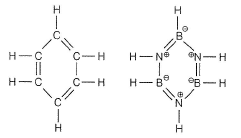(C—H) bond lengths are different from (B—H) and (N—H) bond lengths. Both are planar.

QUESTION: 2

### Which is not the correct structure of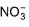ion?

Solution:

In structure (a), octet of N-atom is not complete. In all the other structures, octets of N and all the O-atoms are complete.

QUESTION: 3

### Which of the following angle corresponds to sp2 hybridisation?

Solution:

sp2 hybridisation gives three sp2 hybrid orbitals which are planar triangular forming an angle of 120° with each other.
The electronic configurations of three elements A, B and C are given below.
Answer the questions from 14 to 17 on the basis of these configurations.
A ls22s22p6
B ls22s22p63s23p3
C ls22s22p63s23p

QUESTION: 4

Which of the following species has tetrahedral geometry?

Solution: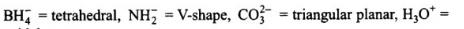pyramidal.

QUESTION: 5

Which of the following structures is more acceptable for HNO3?

Solution:

Structure is decided based on formal charge.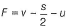where, v = valence electrons
s = shared electrons
u = unshared electrons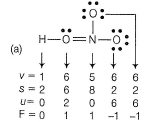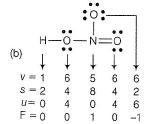Structure with at least one neutral atom is favoured.

QUESTION: 6

Consider the following pairs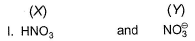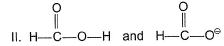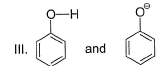Q. More stable species in each pair is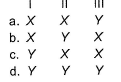Solution:

Greater the resonating structures, greater the stability.
I :(Y)with negative charge has greater delocalisation of n-electrons than HNO3(X).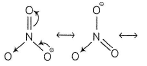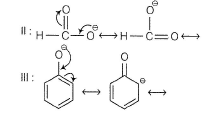QUESTION: 7

Azide ion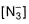exhibits an (N—N) bond order of 2 and may be represented by resonance structures I, II and III given below :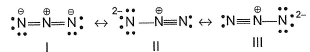Select the correct statement(s) about more contributions,

Solution:

Structure with least formal charge on each atom, makes greater contributions. Also, structures with at least one neutral atom is also favoured II and III identical.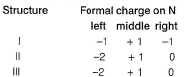QUESTION: 8

C—Cl bond in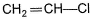(vinyl chloride) is stabilised in the same way as in

Solution: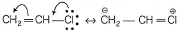Due to delocalisation of π-electrons, (C—Cl) bond is stable and it does not show SN reactions; Cl directly attached (C=C) bond, i.e. vinyl group.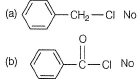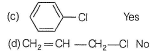QUESTION: 9

NO2 can be represented as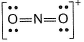Q. Formal charge on each oxygen atom is

Solution:

Formal charge (F)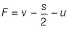where, v = valence electrons = 6
s = shared electrons = 4
u = unshared electron = 4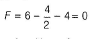QUESTION: 10

Which representation for the Lewis structure of HNO3 is correct?

Solution:

Only structure a is able to show the valence electrons along with charge distributions. So, ith the Lewis structure of HNO3 .

QUESTION: 11

By SN 1 reaction allyl chloride changes to allyl alcohol as shown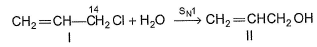Select the correct product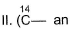isotope of carbon)

Solution:

SN1 means unimolecular (1) nucleophilic (N) substitution (S). Rate is independent of molar concentration of H2O but is dependend on molar concentration of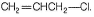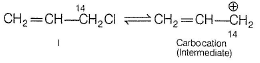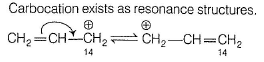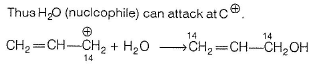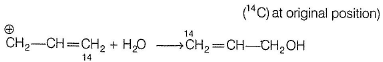(14C at changed position due to resonance).

QUESTION: 12

Stability of (C—Cl) bond in chlorobenzene is sim ilar to (C—Cl) bond in

Solution: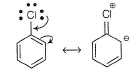(C—Cl) bond has (C=Cl) double bond character, thus it is stable.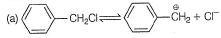Benzyl carbocation is stable due to resonance, hence (C—Cl) bond is cleaved easily.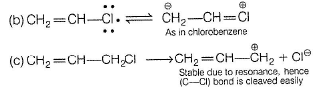QUESTION: 13

Consider the following compounds

I. Vinyl chloride
II. B3N3H6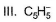Delocalisation takes place

Solution: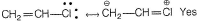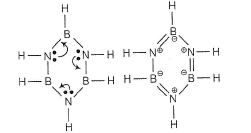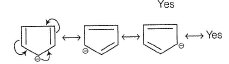QUESTION: 14

Hydrogen bonds are formed in many compounds e.g., H2O, HF, NH3. The boiling point of such compounds depends to a large extent on the strength of hydrogen bond and the number of hydrogen bonds. The correct decreasing order of the boiling points of above compounds is :

Solution:

Strength of H-bonding depends on the electronegativity of the atom which follows the order: F > O > N .
Strength of H-bond is in the order:
H……. F > H…….. O > H…….. N
But each H2O molecule is linked to 4 other H2O molecules through H-bonds whereas each HF molecule is linked only to two other HF molecules.
Hence, correct decreasing order of the boiling points is HzO > HF > NH3.

QUESTION: 15

Out of the following two structures of N2O, which is more accurate?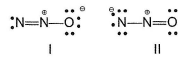Solution:

Plan Oxygen is more electronegative than oxygen, hence the structure with a negative formal charge on oxygen atom is probably lower in energy than the structure that has a negative formal charge on N-atom is more accurate.
Thus (I) is more accurate than (II).

*Multiple options can be correct
QUESTION: 16

Direction (Q. Nos. 16-17) This section contains 4 multiple choice questions. Each question has four choices (a), (b), (c) and (d), out of which ONE or  MORE THANT ONE  is correct.

Q. Select the correct statements about O3.

Solution:

(a) All (O—O) bonds in O3 are equivalent, thus it is resonance hybrid of I and II- True.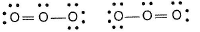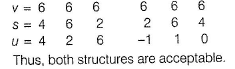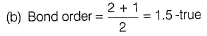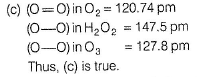(d) No unpaired electron in O3 -diamagnetic.
Thus, (d) is false.

*Multiple options can be correct
QUESTION: 17

Select the correct statement(s) about benzene (C6H6),

Solution:

Due to complete-delocalisation of π-electrons, benzene exists as resonance structures.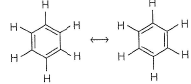Thus, (C=C) bonds and (C —C) bonds are of equal length and stability.
All (C— H) bonds are equivalent.
C-atom is also sp2-hybridised.

QUESTION: 18

Direction (Q. Nos. 18-20) This section contains  a paragraph, each describing theory, experiments, data etc. three Questions related to paragraph have been given.Each question have only one correct answer among the four given options (a),(b),(c),(d).

Fluorine nitrate,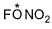is an oxidising agent, used as a rocket propellant.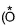this oxygen is different from other two)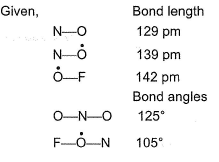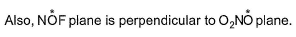Q. Possible structure of FONO2 is

Solution: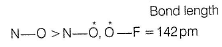double bond single bond character character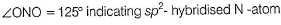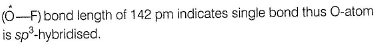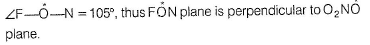QUESTION: 19

Fluorine nitrate,is an oxidising agent, used as a rocket propellant.this oxygen is different from other two)Q. N-atom is ...... hybridised.

Solution:double bond single bond character characterQUESTION: 20

Fluorine nitrate,is an oxidising agent, used as a rocket propellant.this oxygen is different from other two)Q. Which is/are correct a -bonding scheme?

Solution:double bond single bond character character*Answer can only contain numeric values
QUESTION: 21

Direction (Q. Nos. 21 and 22) This section contains 2 questions. when worked out will result in an integer from 0 to 9 (both inclusive).

Q. How many electrons are delocalised in pyridine?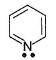Solution:

Lone pair on N-atom is not delocalised in pyridine.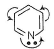Thus total = 6π-electrons.

*Answer can only contain numeric values
QUESTION: 22

Addition of HBr at position A and B in the following compound is called x,y-addition.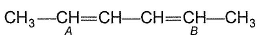Q. What is value of (x + y)?

Solution:

Addition in conjugated diene can be 1 ,2 - or 1 , 4-typ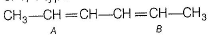At position A,B it is called 1 , 4-addition.